# How to Create Radar Charts in R?

• Last Updated : 22 Feb, 2022

In this article, we are going to see how to create Radar Charts in R Programming Language.

Radar charts are also known as Spider or Web or Polar charts. It is a graphical graph to display multivariate data in from of 2D charts of three or more quantitative variables which are represented on axes starting from the same point

### Dataset in use:

Mumbai Tamil Noida Kerala Patna Assam Ranchi Bhopal Delhi Indore

1     39    39    39    39    39    39     39     39    39     39

2      0     0     0     0     0     0      0      0     0      0

3      2     7     5     5     9    18      7      7    10     19

fmsb package is Several utility functions and it has radarchar() methods to create radar chart in R.

To install the package:

`install.packages( fmsb )`

## Creating a Basic Radar chart in R

Here we will display the basic radar chart using radarchart() methods. For this, we will pass the created dataframe.

Syntax: radarchart(df, cglty, cglcol, cglwd, pcol, plwd)

Parameters:

• df is a dataframe
• pcol attributes is used to change the color of the Polygon line.
• plwd attributes is used to increase or decrease the line width of the Polygon.
• cglcol is used to draw color of the net
• cglwd is for net width

## R

 `data <- ``as.data.frame``(``matrix``( ``sample``( 2:20 , 10 ,` `                                     ``replace=T) ,` `                             ``ncol=10))`   `colnames``(data) <- ``c``(``"Mumbai"` `, ``"Tamil"` `, ``"Noida"` `,` `                    ``"Kerala"` `, ``"Patna"``, ``"Assam"` `,` `                    ``"Ranchi"` `, ``"Bhopal"``, ``"Delhi"``, ` `                    ``"Indore"` `)` ` `  `data <- ``rbind``(``rep``(39,10) , ``rep``(0,10) , data)`   `# Library` `library``(fmsb)`   `# The default radar chart ` `radarchart``(data)`

Output: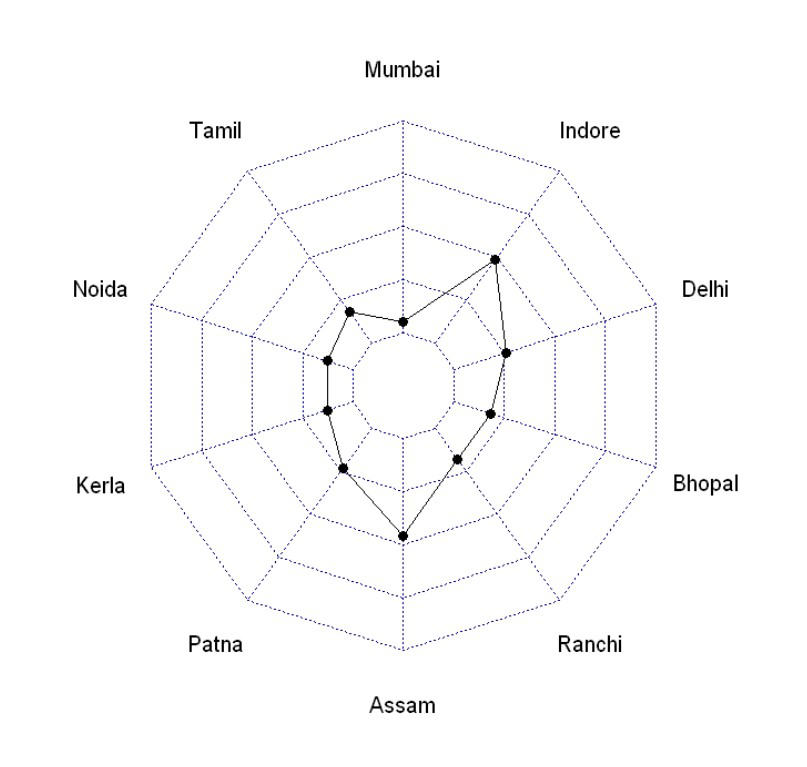## Line Color Customization

pcol attributes are used to change the color of the Polygon line.

Example: Line color customization

## R

 `data <- ``as.data.frame``(``matrix``( ``sample``( 2:20 , 10 ,` `                                     ``replace=T) ,` `                             ``ncol=10))`   `colnames``(data) <- ``c``(``"Mumbai"` `, ``"Tamil"` `, ``"Noida"` `,` `                    ``"Kerala"` `, ``"Patna"``, ``"Assam"` `,` `                    ``"Ranchi"` `, ``"Bhopal"``, ``"Delhi"``, ` `                    ``"Indore"` `)` ` `  `data <- ``rbind``(``rep``(39,10) , ``rep``(0,10) , data)`   `radarchart``(data, pcol = ``"Green"``)`

Output: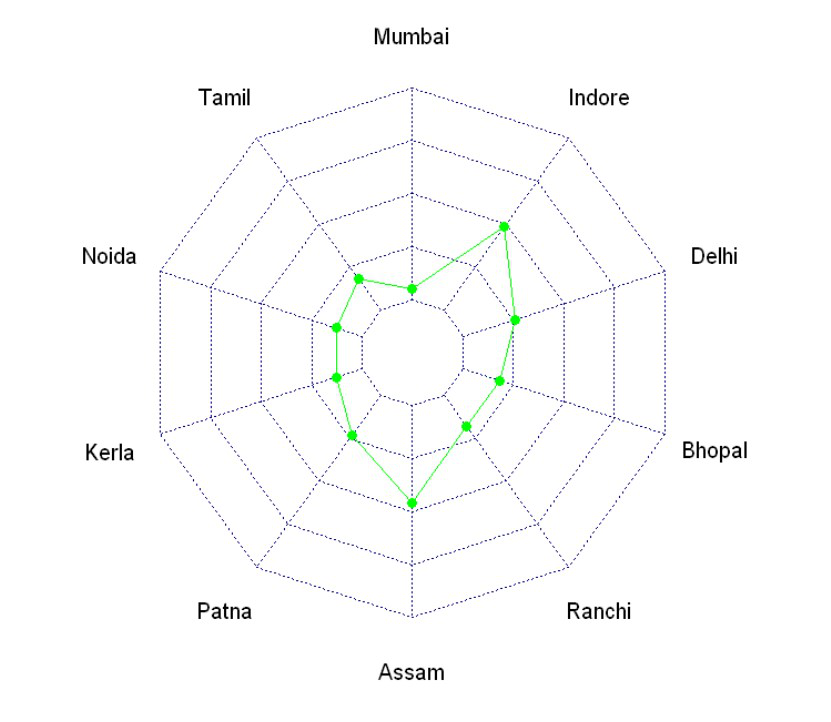## Fill Color into Polygon

pfcol attributes is used to fill the color of the Polygon.

Example: Fill color

## R

 `data <- ``as.data.frame``(``matrix``( ``sample``( 2:20 , 10 ,` `                                     ``replace=T) ,` `                             ``ncol=10))`   `colnames``(data) <- ``c``(``"Mumbai"` `, ``"Tamil"` `, ``"Noida"` `,` `                    ``"Kerala"` `, ``"Patna"``, ``"Assam"` `,` `                    ``"Ranchi"` `, ``"Bhopal"``, ``"Delhi"``, ` `                    ``"Indore"` `)` ` `  `data <- ``rbind``(``rep``(39,10) , ``rep``(0,10) , data)`   `# Library` `library``(fmsb)`   `radarchart``(data, pfcol = ``"Green"``)`

Output: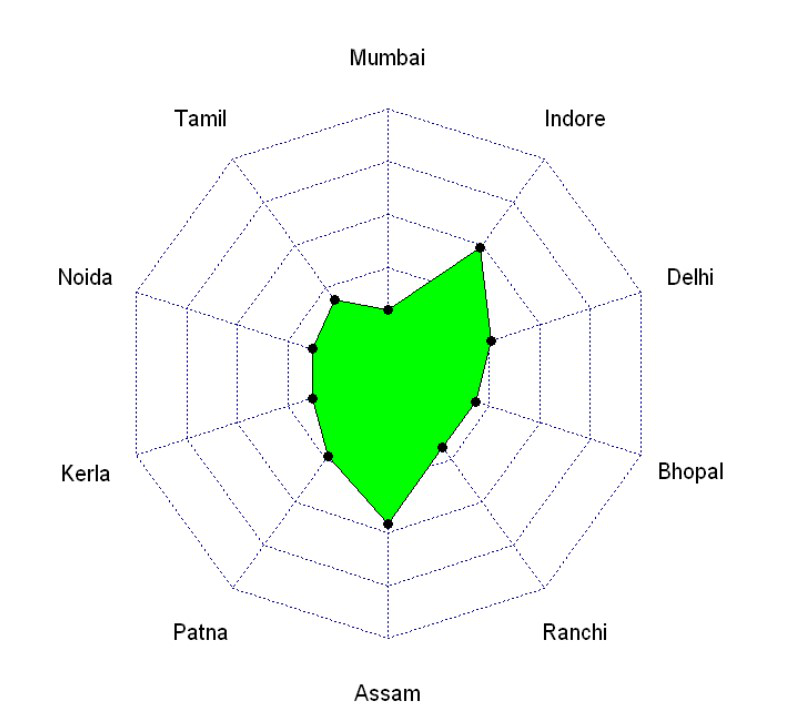## Change the line width

plwd attributes is used to increase or decrease the line width of the Polygon.

Example: Change line width

## R

 `data <- ``as.data.frame``(``matrix``( ``sample``( 2:20 , 10 ,` `                                     ``replace=T) ,` `                             ``ncol=10))`   `colnames``(data) <- ``c``(``"Mumbai"` `, ``"Tamil"` `, ``"Noida"` `,` `                    ``"Kerala"` `, ``"Patna"``, ``"Assam"` `,` `                    ``"Ranchi"` `, ``"Bhopal"``, ``"Delhi"``, ` `                    ``"Indore"` `)` ` `  `data <- ``rbind``(``rep``(39,10) , ``rep``(0,10) , data)`   `# Library` `library``(fmsb)`   `radarchart``(data, plwd = 3)`

Output: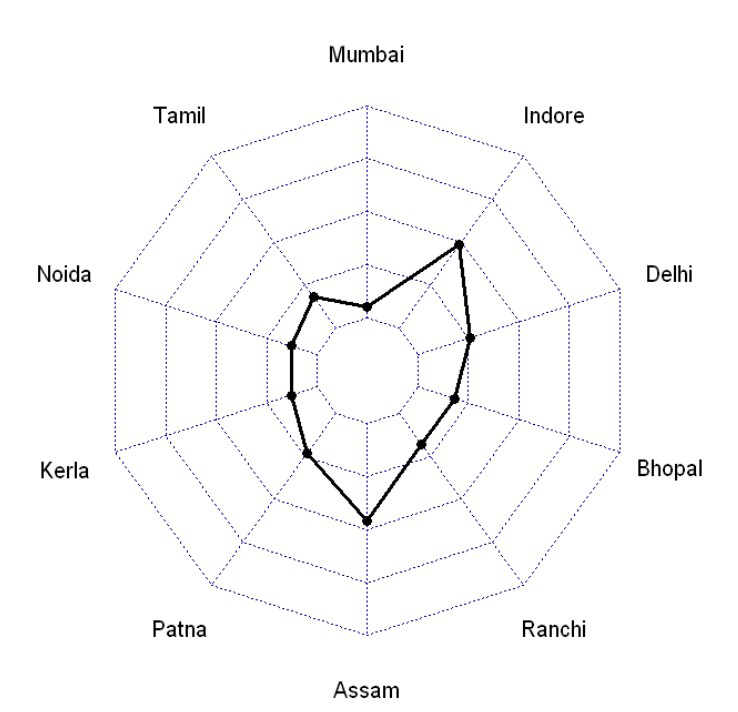Following attributes are used for Grids:

• cglcol is used to draw the color of the net
• cglty is for get  net line type
• axislabcol is for the color of axis labels
• caxislabels is for the vector of axis labels to display
• cglwd is for net width

Example: Grid features

## R

 `data <- ``as.data.frame``(``matrix``( ``sample``( 2:20 , 10 ,` `                                     ``replace=T) ,` `                             ``ncol=10))`   `colnames``(data) <- ``c``(``"Mumbai"` `, ``"Tamil"` `, ``"Noida"` `,` `                    ``"Kerala"` `, ``"Patna"``, ``"Assam"` `,` `                    ``"Ranchi"` `, ``"Bhopal"``, ``"Delhi"``, ` `                    ``"Indore"` `)` ` `  `data <- ``rbind``(``rep``(39,10) , ``rep``(0,10) , data)`   `# Library` `library``(fmsb)`   `radarchart``(data, ` `           ``cglcol=``"Blue"``, cglty=10, axislabcol=``"grey"``,` `           ``caxislabels=``seq``(0,20,5), cglwd=0.8)`

Output: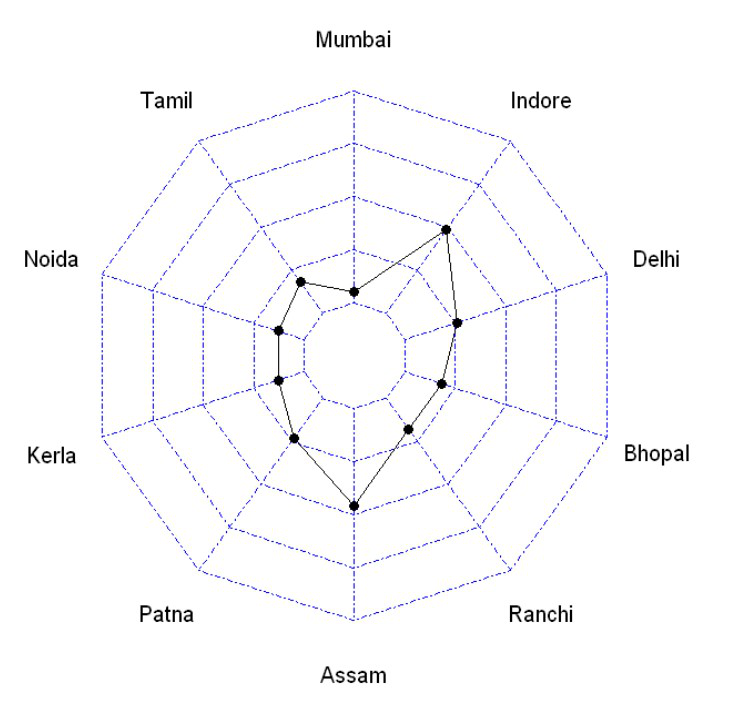My Personal Notes arrow_drop_up
Recommended Articles
Page :